# face detection[PyramidBox]

### 0 引言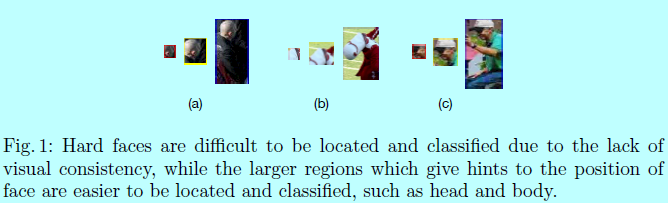• 首先，网络应该学习不止人脸的特征，而且还有包含头和身体的上下文部分。为了达到这个目的，需要额外的标签，并且也需要设计对应的锚。本文中，作者使用了半监督方法去生成人脸上下文部分的相似标签和一系列锚，这里称其为Pyramid Anchors，且该锚也很容易加入到普通基于锚的结构中。
• 然后，high-level的上下文特征应该与low-level的特征适当结合起来。难检测和易检测的人脸外观通常是不同的，这意味着不是所有的high-level语义特征都对更小目标的检测有帮助。作者调研了特征金字塔网络(feature pyramid network)并将其修改为low-level特征金字塔网络（low-level feature pyramid network），以将相互有作用的特征聚合在一起。
• 预测的分支网络应该充分使用联合的特征。这里引入上下文敏感预测模块（context-sensitive prediction module，CPM）并与一个更宽更深的网络相结合，从而能够合并目标人脸周围的上下文信息。同时，在预测模块上提出一个max-in-out层，以此提升分类网络的能力。
• 提出一个训练策略叫data-anchor-sampling，调整了训练数据集上的分布。并通过数据增强的方式来增加难检测样本的多样性，以此获取更具表征的特征。

• 提出一个基于锚的上下文辅助方法，PyramidAnchors，在为了检测小型，模糊和遮挡人脸上引入有监督信息去学习上下文特征；
• 设计一个low-level的特征金字塔网络，更好的融合上下文特征和人脸特征。同时提出的方法可以在单次前向传播中很好的处理不同尺度的人脸；
• 引入一个上下文敏感预测模块，由一个混合网络结构和max-in-out层组成，以从融合的特征中学习准确的位置和类别；
• 提出一个尺度敏感数据-锚-采样策略，改变训练样本的分布，更注重小型人脸；
• 在FDDB和WIDER FACE数据集上获得最好的效果。

### 1 结构

#### 1.1 网络结构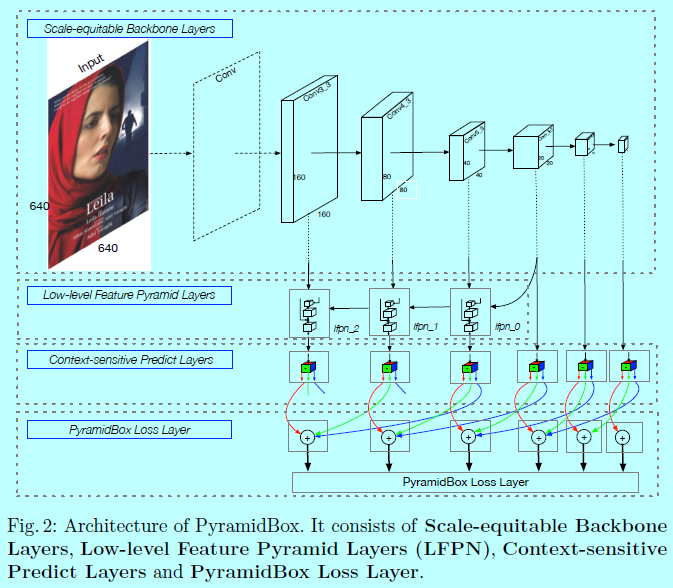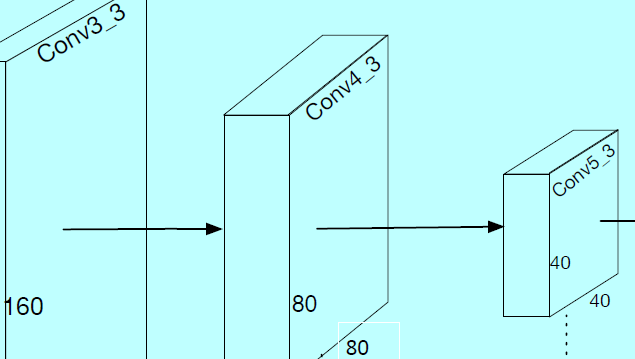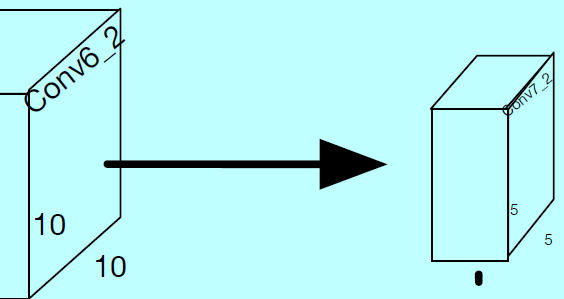PyramidBox的结构也使用VGG16作为基底骨干网络，如$S^3FD$一样的锚尺度设计，即在不同层级上生成feature map和等比例间隔的锚。低层级FPN加在该基底骨干网络上，然后上下文敏感预测模块作为每个金字塔检测层的一个分支网络，从而得到最终的输出。关键在于作者设计了一个新颖的金字塔锚方法，能够在不同level的每个人脸上生成一系列锚。

• 首先，人脸都是很小的，模糊的，遮挡的，不同于那些大的，清晰的，完整的人脸纹理。所以简单的直接将所有high-level特征拿去做小型人脸检测的辅助增强是不可取的；
• 其次，high-level特征部分基本没有了人脸纹理信息，同时还会引入噪音信息。例如，在本文的PyramidBox的基底层中，顶层两层conv7_2和conv6_2的感受野分别是724和468。注意到输入的训练图片大小是640，这意味着顶层两层包含太多噪音上下文特征，所以对于检测中型和小型人脸就没多大帮助。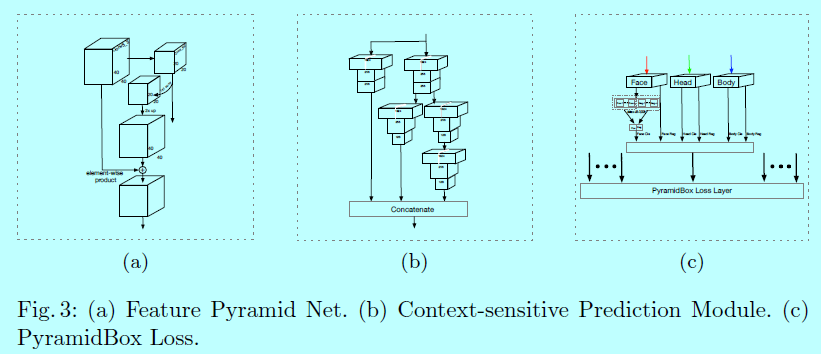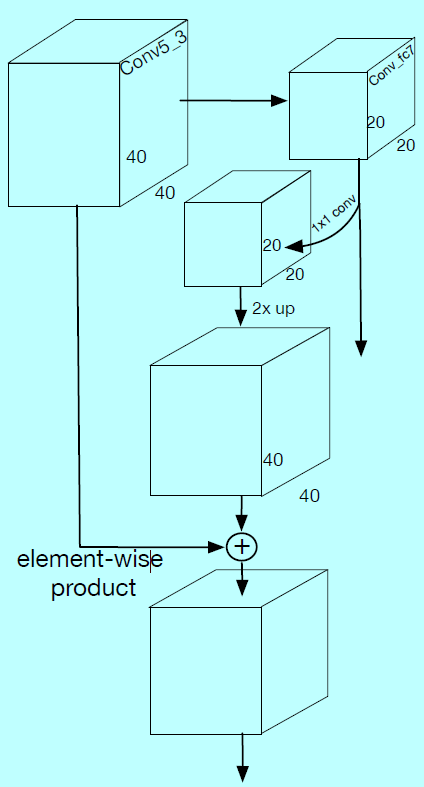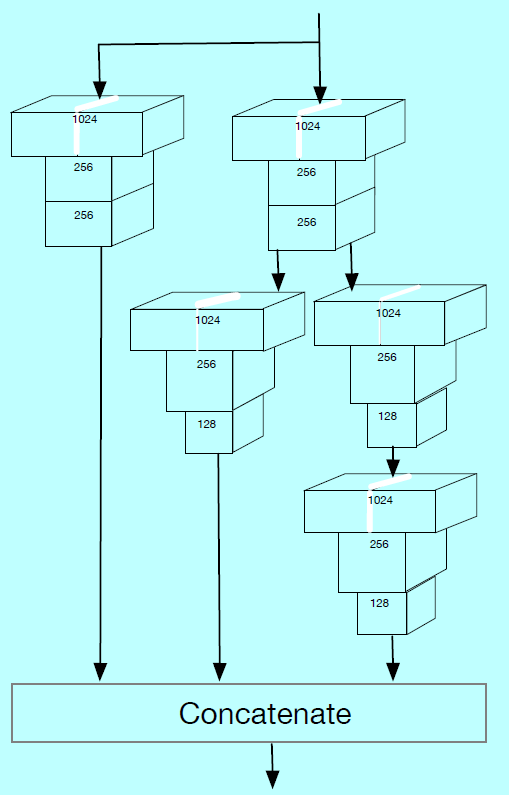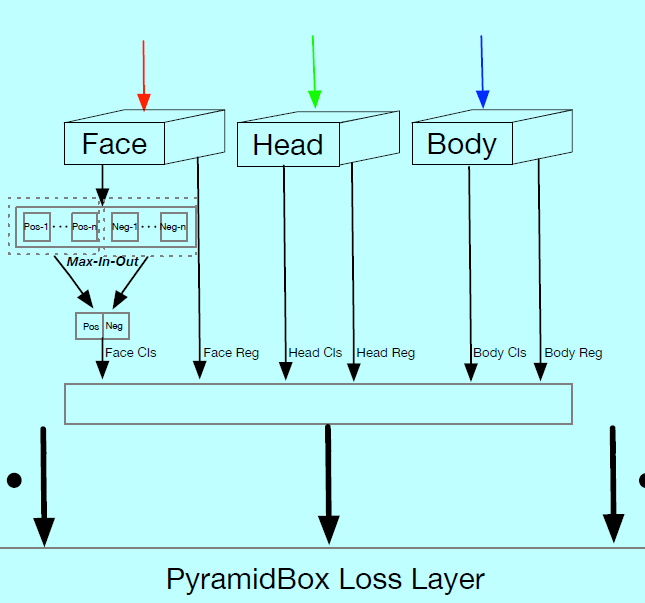$cp_l=\begin{cases} 1, & \text{if } l=0, \\ 3, & otherwise \end{cases}$

PyramidBox loss层

#### 1.2 上下文敏感预测模块

max-in-out
Maxout的概念首次是被GoodFellow提出的，$S^3FD$应用max-out背景标签去减少小的负样本的假阳性比例。在本文中，作者在正样本和负样本上都采用了该方法，称其为max-in-out，如图3c。首先对每个预测模型预测$c_p+c_n$得分，然后选择$\max c_p$作为正得分。相似的，选择$\max c_n$作为负得分。本实验中，因为小型人脸有更多完整的背景，第一个预测模块中$c_p=1$,$c_n=3$，而对于其他预测模块为了召回更多人脸，$c_p=3$,$c_n=1$

#### 1.3 金字塔锚 PyramidAnchors

$label_k(anchor_{i,j})=\begin{cases} 1, & \text{if iou(anchor_{i,j}\cdot s_i/s_{pa}^k, region_{target})>threshold}, \\ 0, & \text{otherwise} \end{cases}$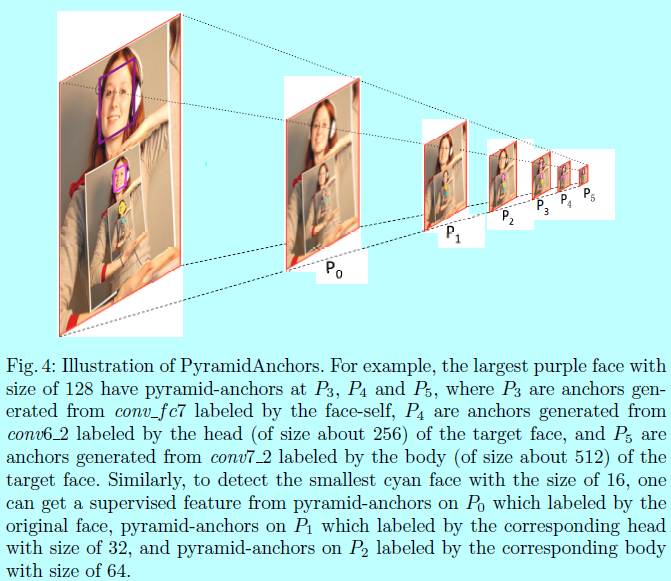#### 1.4 训练

WIDER FACE，包含了颜色失真，随机裁剪，和水平翻转

data-anchor-sampling

$s_i=2^{4+i},for\, i=0,1,...,5. 这里i_{anchor}=argmin_iabs(s_{anchor_i}-s_{face})表示基于选定的人脸最接近的锚的尺度，然后从下面集合中随机选择一个索引i_{target}: \{0,1,...,min(5,i_{anchor}+1)\}$

$s_{target}=random(\frac{s_{i_{target}}}{2}, s_{i_{target}}*2)$

$s^*=s_{target}/s_{face}$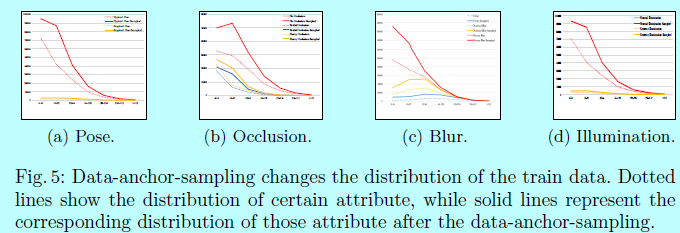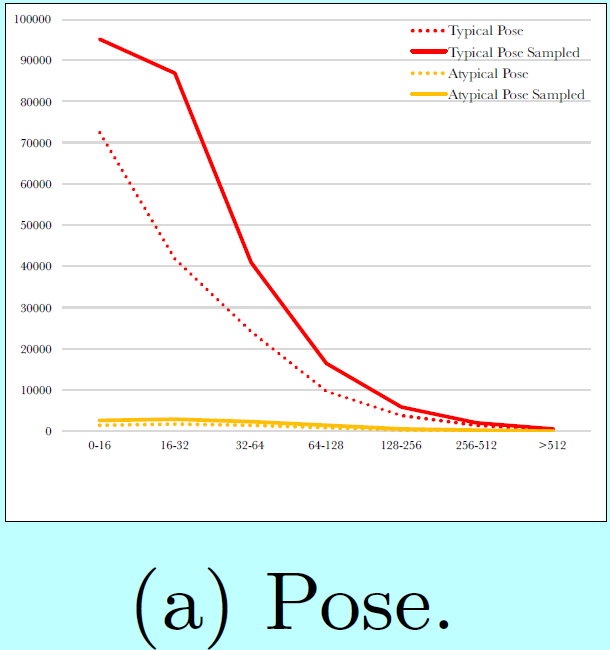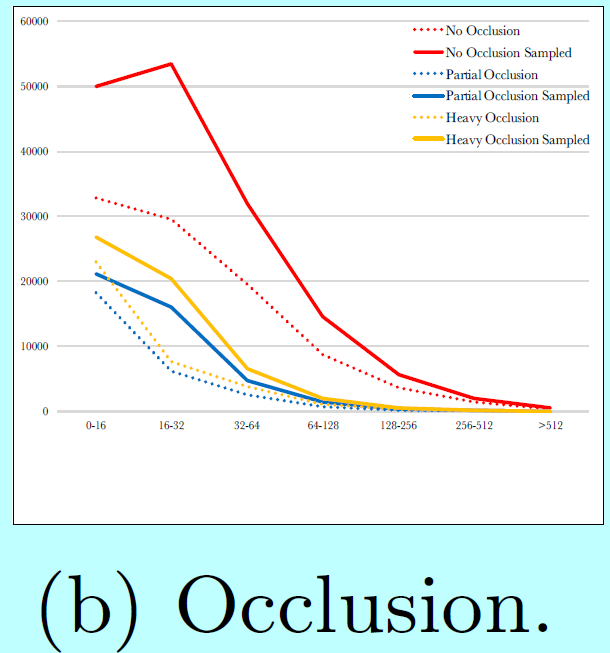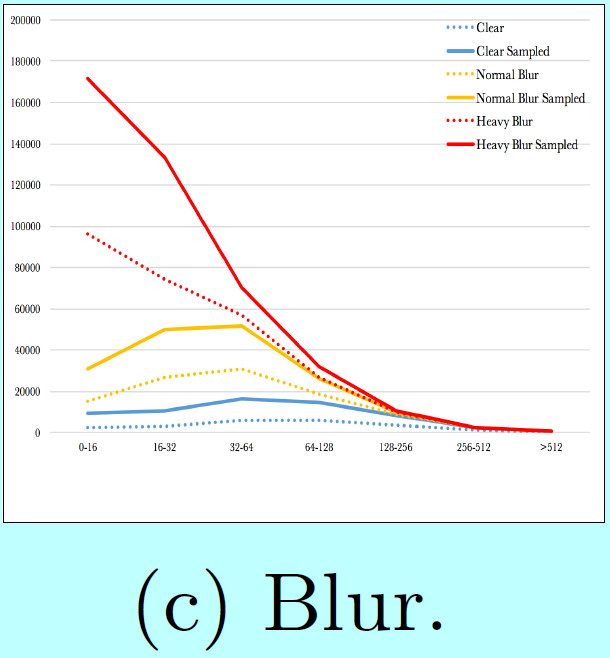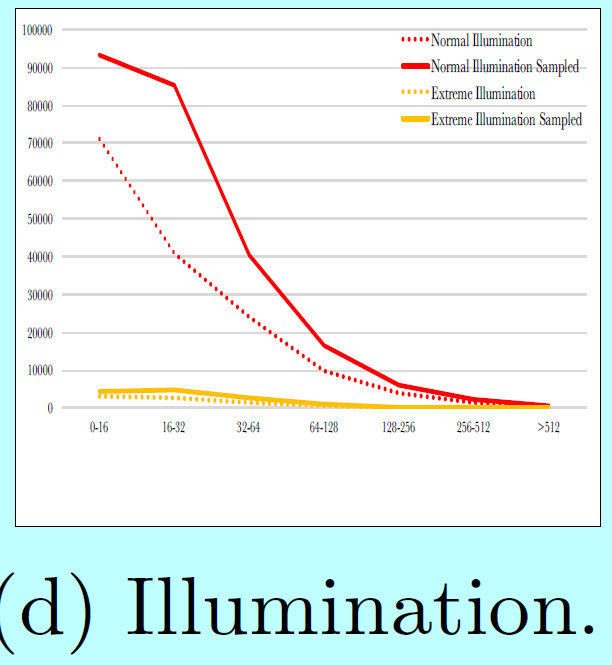data-anchor-sampling改变了训练数据集的分布：

• 小型人脸的比例大于大型人脸的；
• 通过大型人脸中生成小型人脸，增加了更小尺度人脸样本的多样性

PyramidBox Loss

$L(\{p_{k,i}\},\{t_{k,i}\})=\sum_k\lambda_kL_k(\{p_{k,i}\},\{t_{k,i}\})$

$L_k(\{p_{k,i}\},\{t_{k,i}\})=\frac{\lambda}{N_{k,cls}}\sum_{i_k}L_{k,cls}(p_{k,i},p_{k,i}^*)+\frac{1}{N_{k,reg}}\sum_{i_k}p_{k,i}^*\, L_{k,reg}\, (t_{k,i},t_{k,i}^*)$

$p_{k,i}^* = \begin{cases} 1, & \text{if the anchor down-sampled by stride s_{pa}^k is positive;} \\ 0, & \text{otherwise} \end{cases}$

$t_{k,i}^*=(t_x^*+\frac{1-s_{pa}^k}{2}t_w^*s_{w,k}+\Delta_{x,k},\, t_y^*+\frac{1-s_{pa}^k}{2}t_h^*s_{h,k}+\Delta_{y,k},\, s_{pa}^kt_w^*s_{w,k}-2\Delta_{x,k},\, s_{pa}^kt_h^*s_{h,k}-2\Delta_{y,k})$

• $k<2$时，$\Delta_{x,k}=\Delta_{y,k}=0$,$s_{w,k}=s_{h,k}=1$
• $k=2$时，$\Delta_{x,2}=0, \Delta_{y,2}=t_h^*$,$s_{w,2}=\frac{7}{8},s_{h,2}=1$

.

posted @ 2018-11-30 09:09  仙守  阅读(2333)  评论(0编辑  收藏  举报